# pylops.signalprocessing.Convolve1D¶

class pylops.signalprocessing.Convolve1D(N, h, offset=0, dims=None, dir=0, dtype='float64', method=None)[source]

1D convolution operator.

Apply one-dimensional convolution with a compact filter to model (and data) along a specific direction of a multi-dimensional array depending on the choice of dir.

Parameters: N : int Number of samples in model. h : numpy.ndarray 1d compact filter to be convolved to input signal offset : int Index of the center of the compact filter dims : tuple Number of samples for each dimension (None if only one dimension is available) dir : int, optional Direction along which convolution is applied method : str, optional Method used to calculate the convolution (direct, fft, or overlapadd). Note that only direct and fft are allowed when dims=None, whilst fft and overlapadd are allowed when dims is provided. dtype : str, optional Type of elements in input array. ValueError If offset is bigger than len(h) - 1 NotImplementedError If method provided is not allowed

Notes

The Convolve1D operator applies convolution between the input signal $$x(t)$$ and a compact filter kernel $$h(t)$$ in forward model:

$y(t) = \int\limits_{-\infty}^{\infty} h(t-\tau) x(\tau) \,\mathrm{d}\tau$

This operation can be discretized as follows

$y[n] = \sum_{m=-\infty}^{\infty} h[n-m] x[m]$

as well as performed in the frequency domain.

$Y(f) = \mathscr{F} (h(t)) * \mathscr{F} (x(t))$

Convolve1D operator uses scipy.signal.convolve that automatically chooses the best domain for the operation to be carried out for one dimensional inputs. The fft implementation scipy.signal.fftconvolve is however enforced for signals in 2 or more dimensions as this routine efficently operates on multi-dimensional arrays.

As the adjoint of convolution is correlation, Convolve1D operator applies correlation in the adjoint mode.

In time domain:

$x(t) = \int\limits_{-\infty}^{\infty} h(t+\tau) x(\tau) \,\mathrm{d}\tau$

or in frequency domain:

$y(t) = \mathscr{F}^{-1} (H(f)^* * X(f))$
Attributes: shape : tuple Operator shape explicit : bool Operator contains a matrix that can be solved explicitly (True) or not (False)

Methods

 __init__(N, h[, offset, dims, dir, dtype, …]) Initialize this LinearOperator. adjoint() Hermitian adjoint. apply_columns(cols) Apply subset of columns of operator cond([uselobpcg]) Condition number of linear operator. conj() Complex conjugate operator div(y[, niter]) Solve the linear problem $$\mathbf{y}=\mathbf{A}\mathbf{x}$$. dot(x) Matrix-matrix or matrix-vector multiplication. eigs([neigs, symmetric, niter, uselobpcg]) Most significant eigenvalues of linear operator. matmat(X) Matrix-matrix multiplication. matvec(x) Matrix-vector multiplication. rmatmat(X) Matrix-matrix multiplication. rmatvec(x) Adjoint matrix-vector multiplication. todense([backend]) Return dense matrix. toimag([forw, adj]) Imag operator toreal([forw, adj]) Real operator tosparse() Return sparse matrix. trace([neval, method, backend]) Trace of linear operator. transpose() Transpose this linear operator.

## Examples using pylops.signalprocessing.Convolve1D¶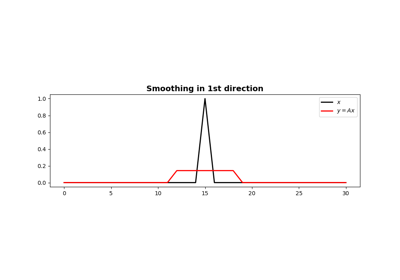1D Smoothing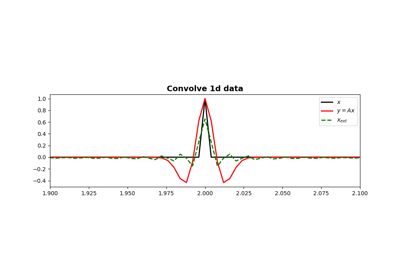Convolution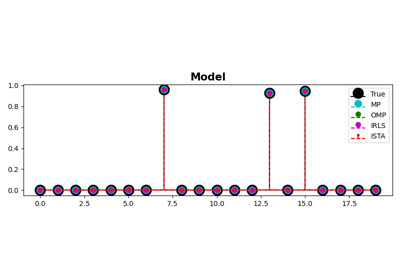MP, OMP, ISTA and FISTA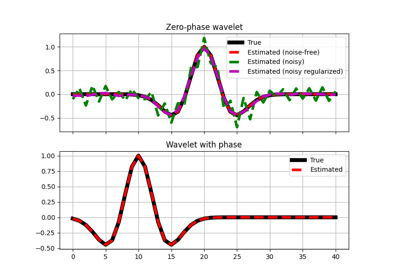Wavelet estimation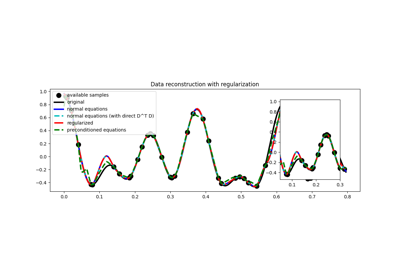03. Solvers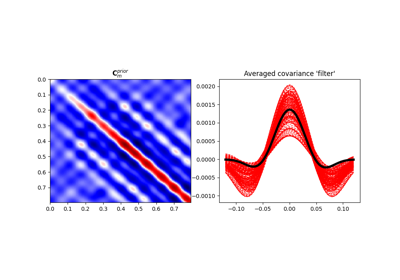04. Bayesian Inversion